# In carbon compounds, the carbon atom can achieve an octet configuration in several ways. Draw the Lewis structure of a hydrocarbon compound in which: (a) Carbon is bonded via four single bonds to adjacent atoms, (b) Carbon is bonded via two single bonds and one pi bond; (c) Carbon is bonded via one single bond and one triple bond; (d) Carbon is bonded via two double bonds.### Chemistry & Chemical Reactivity

10th Edition
John C. Kotz + 3 others
Publisher: Cengage Learning
ISBN: 9781337399074### Chemistry & Chemical Reactivity

10th Edition
John C. Kotz + 3 others
Publisher: Cengage Learning
ISBN: 9781337399074

#### Solutions

Chapter
Section
Chapter 23, Problem 1PS
Textbook Problem

## In carbon compounds, the carbon atom can achieve an octet configuration in several ways. Draw the Lewis structure of a hydrocarbon compound in which: (a) Carbon is bonded via four single bonds to adjacent atoms, (b) Carbon is bonded via two single bonds and one pi bond; (c) Carbon is bonded via one single bond and one triple bond; (d) Carbon is bonded via two double bonds.

Expert Solution

(a)

Interpretation Introduction

Interpretation:

The Lewis structure of organic compound in which Carbon is bonded via four single bonds to adjacent atoms  should be drawn

Concept introduction:

• Lewis structure is also known as Lewis dot structure which represents the bonding between atoms of a molecule and the lone pair of that may exist in the molecule.
• Carbon atomic number 6. Electronic configuration -1s22s22p2.
• Carbon has tetra valency. It is bonded with four bonds to adjacent atoms or molecules.

Single covalent bond - one pair of each electrons are shared.

Double covalent bond – two pair of electrons are shared.

Triple covalent bond – Three pairs of electron are shared.### Explanation of Solution

Carbon is bonded via four single bonds to adjacent atoms. Carbon is bonded with four single four hydrogen atoms.

The Lewis electron dot structure for given molecules are determined by first drawing the skeletal structure for the given molecules, then the total number of valence electrons for all atoms present in the molecules are determined.

The next step is to subtract the electrons present in the total number of bonds present in the skeletal structure of the molecule with the total valence electrons such that considering each bond contains two electrons with it.

Finally, the electrons which got after subtractions have to be equally distributed considering each atom contains eight electrons in its valence shell.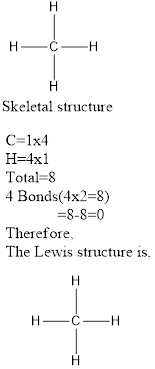Expert Solution

(b)

Interpretation Introduction

Interpretation:

The Lewis structure of organic compound in which carbon is bonded via two single bonds and one pi bond should be drawn

Concept introduction:

• Lewis structure is also known as Lewis dot structure which represents the bonding between atoms of molecules and the lone pair of that may exist in the molecule.
• Carbon atomic number 6. Electronic configuration -1s22s22p2.
• Carbon has tetra valency. It is bonded with four bonds to adjacent atoms or molecules.

Single covalent bond - one pair of each electrons are shared.

Double covalent bond – two pair of electrons are shared.

Triple covalent bond – Three pairs of electron are shared.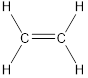### Explanation of Solution

Carbon is bonded via two single bonds and one pi bond.

Side overlapping of the two adjacent carbon atoms of orbitals form pi-bond.

The Lewis electron dot structure for given molecules are determined by first drawing the skeletal structure for the given molecules, then the total number of valence electrons for all atoms present in the molecules are determined.

The next step is to subtract the electrons present in the total number of bonds present in the skeletal structure of the molecule with the total valence electrons such that considering each bond contains two electrons with it.

Finally, the electrons which got after subtractions have to be equally distributed considering each atom contains eight electrons in its valence shell.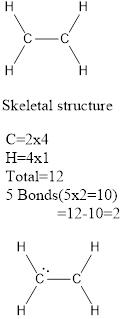Here, the octet rule does not obey for carbon so a double bond is formed between two carbon atoms.

The Lewis structure as shown below.Expert Solution

(c)

Interpretation Introduction

Interpretation:

The Lewis structure of organic compound in which carbon is bonded via one single bond and one triple bond should be drawn

Concept introduction:

• Lewis structure is also known as Lewis dot structure which represents the bonding between atoms of molecules and the lone pair of that may exist in the molecule.
• Carbon atomic number 6. Electronic configuration -1s22s22p2.
• Carbon has tetra valency. It is bonded with four bonds to adjacent atoms or molecules.

Single covalent bond - one pair of each electrons are shared.

Double covalent bond – two pair of electrons are shared.

Triple covalent bond – Three pairs of electron are shared.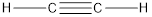### Explanation of Solution

Carbon is bonded via one single bond and one triple bond. Three pairs of electron are shared by two adjacent carbon atoms.

The Lewis electron dot structure for given molecules are determined by first drawing the skeletal structure for the given molecules, then the total number of valence electrons for all atoms present in the molecules are determined.

The next step is to subtract the electrons present in the total number of bonds present in the skeletal structure of the molecule with the total valence electrons such that considering each bond contains two electrons with it.

Finally, the electrons which got after subtractions have to be equally distributed considering each atom contains eight electrons in its valence shell.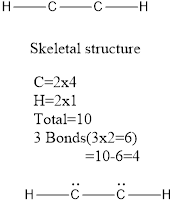Here, the octet rule does not obey for carbon so a triple bond is formed between two carbon atoms.

The Lewis structure as shown below.Expert Solution

(d)

Interpretation Introduction

Interpretation:

The Lewis structure of organic compound in which carbon is bonded via two double bonds should be drawn

Concept introduction:

• Lewis structure is also known as Lewis dot structure which represents the bonding between atoms of a molecules and the lone pair of that may exist in the molecule.
• Carbon atomic number 6. Electronic configuration -1s22s22p2.
• Carbon has tetra valency. It is bonded with four bonds to adjacent atoms or molecules.

Single covalent bond - one pair of each electrons are shared.

Double covalent bond – two pair of electrons are shared.

Triple covalent bond – Three pairs of electron are shared.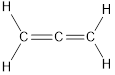### Explanation of Solution

Carbon is bonded via two double bonds. The three adjacent carbon atoms orbitals overlap to form two pi bonds.

The Lewis electron dot structure for given molecules are determined by first drawing the skeletal structure for the given molecules, then the total number of valence electrons for all atoms present in the molecules are determined.

The next step is to subtract the electrons present in the total number of bonds present in the skeletal structure of the molecule with the total valence electrons such that considering each bond contains two electrons with it.

Finally, the electrons which got after subtractions have to be equally distributed considering each atom contains eight electrons in its valence shell.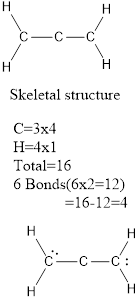Here, the octet rule does not obey for carbon so two double bond are formed between the carbon atoms.

The Lewis structure as shown below.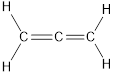### Want to see more full solutions like this?

Subscribe now to access step-by-step solutions to millions of textbook problems written by subject matter experts!

Get Solutions

### Want to see more full solutions like this?

Subscribe now to access step-by-step solutions to millions of textbook problems written by subject matter experts!

Get Solutions